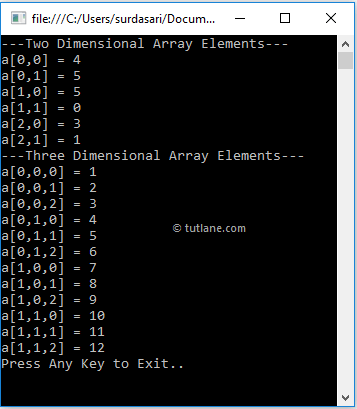# Visual Basic Multidimensional Arrays

As discussed in the previous chapter, Arrays in Visual Basic will support multi-dimensional arrays.  In visual basic, a multidimensional array is an array that contains more than one dimension to represent the elements in a tabular format like rows and columns.

In visual basic, multidimensional arrays can support either two or three-dimensional series. To create multi-dimensional arrays, we need to use comma (,) separator inside of the brackets.

## Visual Basic Multi-Dimensional Array Declaration

In visual basic, Multidimensional Arrays can be declared by specifying the data type of an elements followed by the brackets () with comma (,) separator. Following are the examples of creating two or three-dimensional arrays in visual basic programming language.

' Two Dimensional Array

Dim arr As Integer(,) = New Integer(3, 1) {}

' Three Dimensional Array

Dim arr1 As Integer(,,) = New Integer(3, 1, 2) {}

If you observe the above examples, we created two-dimensional array (arr) with 4 rows, 2 columns and we created another array (arr1) with three dimensions 4, 2, 3.

## Visual Basic Multi-Dimensional Array Initialization

In visual basic, we can initialize arrays upon declaration. Following are the different ways of declaring and initializing the multidimensional arrays in visual basic programming language.

' Two Dimensional Integer Array

Dim intarr As Integer(,) = New Integer(2, 1) {{4, 5}, {5, 0}, {3, 1}}

' Two Dimensional Integer Array without Dimensions

Dim intarr1 As Integer(,) = New Integer(,) {{4, 5}, {5, 0}, {3, 1}}

' Three Dimensional Array

Dim array3D As Integer(,,) = New Integer(1, 1, 2) {{{1, 2, 3}, {4, 5, 6}}, {{7, 8, 9}, {10, 11, 12}}}

' Three Dimensional Array without Dimensions

Dim array3D1 As Integer(,,) = New Integer(,,) {{{1, 2, 3}, {4, 5, 6}}, {{7, 8, 9}, {10, 11, 12}}}

If you observe the above examples, we declared and initialized two dimensional array with 3 rows and 2 columns and three dimensional array with 2, 2, 3 dimensions.

In second and fourth statements, while declaration we initialized arrays with values, but without specifying any dimensions. Here, the dimensions of array can be determined by the number of elements. So, the dimension initializers are not required in case if we are assigning an elements during the initialization.

## Visual Basic Access Multidimensional Array Elements

In visual basic, we can access the values of multidimensional arrays by using a row index and column index values.

Following is the example of accessing the elements of multidimensional arrays in visual basic programming language based on our requirements.

' Two Dimensional Array

Dim arr2D As Integer(,) = New Integer(2, 1) {{4, 5}, {5, 0}, {3, 1}}

' Three Dimensional Array

Dim array3D As Integer(,,) = New Integer(1, 1, 2) {{{1, 2, 3}, {4, 5, 6}}, {{7, 8, 9}, {10, 11, 12}}}

Console.WriteLine(arr2D(1, 0)) ' 5

Console.WriteLine(array3D(1, 1, 1)) ' 11

If you observe the above example, we are accessing the elements of two & three dimensional arrays by passing the index values.

## Visual Basic Multidimensional Array Example

Following is the example of using multidimensional arrays in visual basic programming language to represent the elements in an array with multiple dimensions.

Module Module1

Sub Main()

' Two Dimensional Array

Dim array2D As Integer(,) = New Integer(2, 1) {{4, 5}, {5, 0}, {3, 1}}

' Three Dimensional Array

Dim array3D As Integer(,,) = New Integer(1, 1, 2) {{{1, 2, 3}, {4, 5, 6}}, {{7, 8, 9}, {10, 11, 12}}}

Console.WriteLine("---Two Dimensional Array Elements---")

For i As Integer = 0 To 3 - 1

For j As Integer = 0 To 2 - 1

Console.WriteLine("a[{0},{1}] = {2}", i, j, array2D(i, j))

Next

Next

Console.WriteLine("---Three Dimensional Array Elements---")

For i As Integer = 0 To 2 - 1

For j As Integer = 0 To 2 - 1

For k As Integer = 0 To 3 - 1

Console.WriteLine("a[{0},{1},{2}] = {3}", i, j, k, array3D(i, j, k))

Next

Next

Next

Console.WriteLine("Press Enter Key to Exit..")

End Sub

End Module

If you observe the above example, we created two and three dimensional arrays and getting those array values by using for loop in vb.

When we execute the above visual basic program, we will get the result as shown below.If you observe the above result, we are able to get the values of two and three-dimensional arrays by using respective index values based on our requirements.

In visual basic, multidimensional arrays are slower than single dimensional arrays while accessing the elements from arrays. Instead of multidimensional arrays, we can use jagged arrays in c# to improve the performance of the application.

This is how we can use the multidimensional arrays in our visual basic applications based on our requirements.